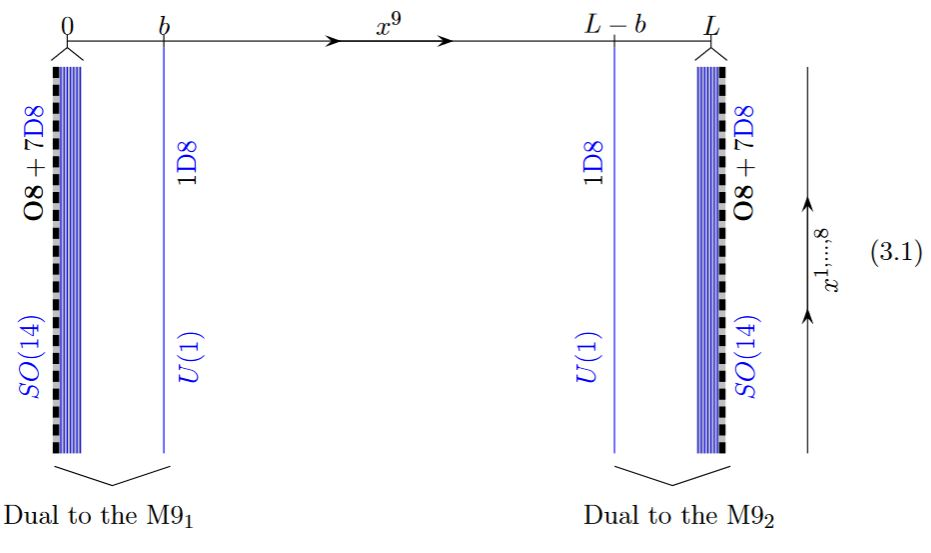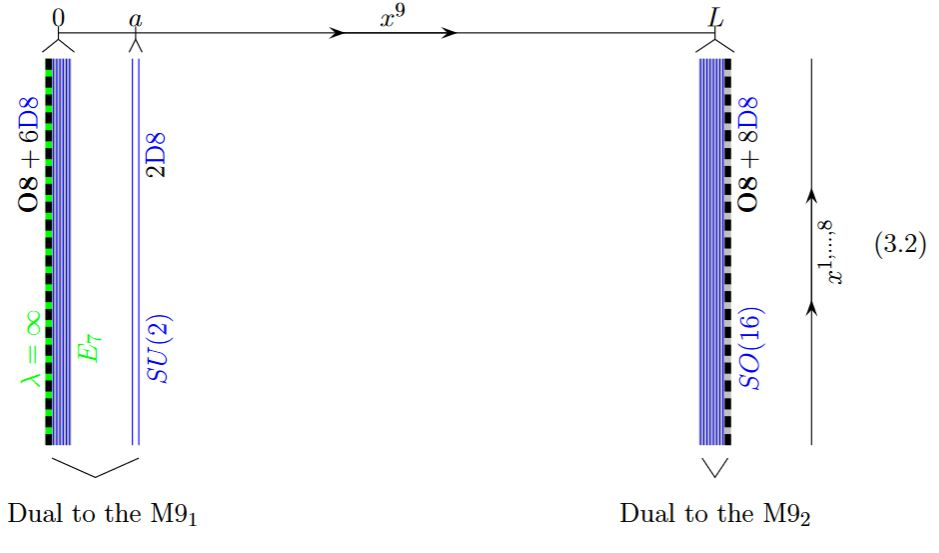Contents

Idea

The $\mathbb{Z}_2$-orbifold/higher orientifold fixed point in Hořava-Witten theory might be called it O9-plane but is often called the M9-brane (e.g. GKSTY 02, section 3, Moore-Peradze-Saulina 04), even though it is on a different conceptual footing than the genuine M2-brane and M5-brane.

In (Hull 97, pages 8-9) the M9-brane was argued to be the object whose charge is the Poincaré dual to the time-component of the M2-brane charge as it appears in the M-theory super Lie algebra via

$\wedge^2 (\mathbb{R}^{10,1})^\ast \simeq \wedge^2 (\mathbb{R}^{10})^\ast \oplus \wedge^{9} \mathbb{R}^{10} \,.$

(In the same way the time component of the M5-brane charge is argued to be dual to the charge of the KK-monopole, see there.)

Under the duality between M-theory and type IIA string theory the M9-brane may be identified with the O8-plane:This may be used to understand the gauge enhancement to E8-gauge groups at the heterotic boundary of Horava-Witten theory:

ViaO8GaugeEnhancementOnM9.jpgTable of branes appearing in supergravity/string theory (for classification see at brane scan).

branein supergravitycharged under gauge fieldhas worldvolume theory
black branesupergravityhigher gauge fieldSCFT
D-branetype IIRR-fieldsuper Yang-Mills theory
$(D = 2n)$type IIA$\,$$\,$
D(-2)-brane$\,$$\,$
D0-brane$\,$$\,$BFSS matrix model
D2-brane$\,$$\,$$\,$
D4-brane$\,$$\,$D=5 super Yang-Mills theory with Khovanov homology observables
D6-brane$\,$$\,$D=7 super Yang-Mills theory
D8-brane$\,$$\,$
$(D = 2n+1)$type IIB$\,$$\,$
D(-1)-brane$\,$$\,$$\,$
D1-brane$\,$$\,$2d CFT with BH entropy
D3-brane$\,$$\,$N=4 D=4 super Yang-Mills theory
D5-brane$\,$$\,$$\,$
D7-brane$\,$$\,$$\,$
D9-brane$\,$$\,$$\,$
(p,q)-string$\,$$\,$$\,$
(D25-brane)(bosonic string theory)
NS-branetype I, II, heteroticcircle n-connection$\,$
string$\,$B2-field2d SCFT
NS5-brane$\,$B6-fieldlittle string theory
D-brane for topological string$\,$
A-brane$\,$
B-brane$\,$
M-brane11D SuGra/M-theorycircle n-connection$\,$
M2-brane$\,$C3-fieldABJM theory, BLG model
M5-brane$\,$C6-field6d (2,0)-superconformal QFT
M9-brane/O9-planeheterotic string theory
M-wave
topological M2-branetopological M-theoryC3-field on G2-manifold
topological M5-brane$\,$C6-field on G2-manifold
S-brane
SM2-brane,
membrane instanton
M5-brane instanton
D3-brane instanton
solitons on M5-brane6d (2,0)-superconformal QFT
self-dual stringself-dual B-field
3-brane in 6d

Discussion of a possible black 9-brane in 11d is in

The term “M9-brane” maybe originates in

A proposal for a description of the M9 as an higher WZW theory is in

• Takeshi Sato, A 10-form Gauge Potential and an M-9-brane Wess-Zumino Action in Massive 11D Theory, Phys. Lett. B477 (2000) 457-468 (arXiv:hep-th/9912030)

Discussion of how the M2-brane and the M5-brane may arise from this by tachyon condensation is in

• Laurent Houart, Yolanda Lozano, Brane Descent Relations in M-theory, Phys.Lett. B479 (2000) 299-307 (arXiv:hep-th/0001170)

Discussion of the M9 as the dual in Horava-Witten theory of O8-planes in type II string theory is in

• E. Gorbatov, V.S. Kaplunovsky, J. Sonnenschein, Stefan Theisen, S. Yankielowicz, section 3 of On Heterotic Orbifolds, M Theory and Type I’ Brane Engineering, JHEP 0205:015, 2002 (arXiv:hep-th/0108135)